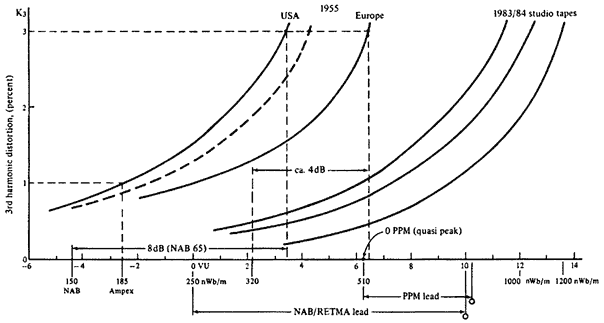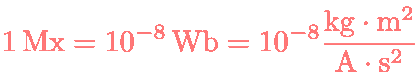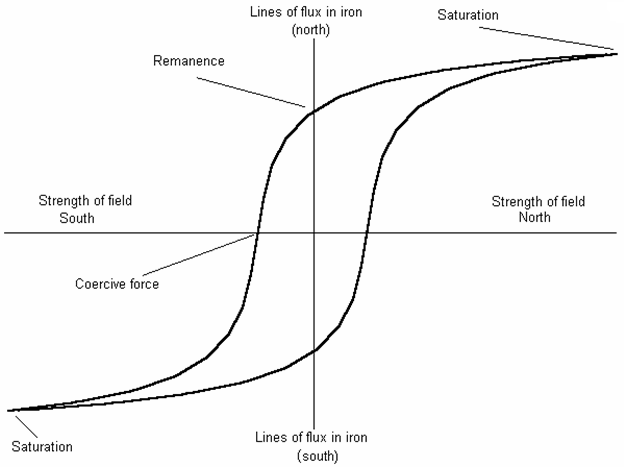Deutsche VersionMagnetic TapeAudio Tape

Conversion: Magnetic flux Φ in nWb ●
(per meter track width) to
flux level in dB

Tape Operating Levels (dB) - Tape Alignment Levels (dB)

 Nanoweber per meter (nWb/m) = picoweber per millimeter (pWb/mm)

 Magnetic flux - most often denoted as Φm. The SI unit of magnetic flux (magnetic field) is the weber (Wb) in derived units: volt-seconds.

 Enter any two values - the third will be calculated.

 For magnetic tapes: Magnetic flux Φ in nWb/m = pWb/mm (tape flux) Fluxivity is the amount of magnetic field that can be stored on a tape.
 Standard reference flux Φ nWb/m Recorded magnetic flux Φ nWb/m Magnetic flux level LΦ dB
 The "magnetic flux density" B is the magnetic flux Φ divided by a surface (area) A: B = Φ / A The magnetic flux density B (magnetic induction) has the unit "weber/m2 (divided by "square meter"). The magnetic flux Φ is not equal to the magnetic flux density B, because the magnetic flux is not divided by meter squared (m2)! We use Wb/m for the track width of the magnetic tape, that is a length measure - and no area. For magnetic tape we do not use the "magnetic flux density", as you often can find.

 The magnetic flux density B in Tesla (T) is not the magnetic flux Φ in Weber (Wb)

 Magnetic    flux Φ Flux level    LΦ 520 nWb/m +8.98 dB 370 nWb/m +6.02 dB 320 nWb/m +4.76 dB 250 nWb/m +2.62 dB 220 nWb/m +1.51 dB 200 nWb/m +0.68 dB 185 nWb/m 0 dB 150 nWb/m −1.82 dB

 Magnetic    flux Φ Flux level    LΦ 520 nWb/m +6.36 dB 370 nWb/m +3.41 dB 320 nWb/m +2.14 dB 250 nWb/m 0 dB 220 nWb/m −1.11 dB 200 nWb/m −1.94 dB 185 nWb/m −2.62 dB 150 nWb/m −4.44 dB

Tape Operating Levels

 Magnetic flux level LΦ = 20 × log (Φ/Φ0) in dB.

Used values as standard reference flux Φ0:
150, 185, 200, 220, 250, 320, 370, or 520 nWb/m.Maximum Output Level (MOL) performance of magnetic recording tapes at 15 ips. O = Theoretical peak flux values when aligning VU meter or PPM. The dashed line (1955) represents the performance of the old US tape if flux values were measured in accordance with DIN. The Maximum Output Level (MOL) is the flux level at which third-harmonic distortion reaches 3% of the fundamental's level measured at 1 kHz (or 5% at 315 Hz for cassettes). There are a number of accepted magnetic reference levels worldwide.

"Maxwell" − the old unit for magnetic flux:tesla (T) − metric system − Unit of magnetic flux density. Unit is named after Nikola Tesla. Gauss (G) − cgs − Unit of magnetic flux density, that is equal to 0.0001 Tesla. Unit is named after Carl Friedrich Gauss. weber/square meter − Unit of magnetic flux density. 1 Wb/m2 = 1 T = 104 Gauss. weber/square centimeter − Unit of magnetic flux density. 1 Wb/cm2 = 10000 T. maxwell/square meter − Unit of magnetic flux density. 1 Mx/m2. maxwell/square centimeter − Unit of magnetic flux density. 1 Mx/cm2. 1 Wb = 108 Mx. and 1 Mx = 10−8 Wb.

 Tape flux: 18.5 mM/mm = 185 nWb/m and 25 mMx/mm = 250 nWb/m

 1 Picoweber per millimeter was 0.1 millimaxwell per millimeterWeber is the practical unit of magnetic flux. It is the amount of magnetic flux which, when linked at a uniform rate with a single-turn electric circuit during an interval of 1 second, will induce in this circuit an electromotive force of 1 volt. Wb = V·s = m2kg/s2A.